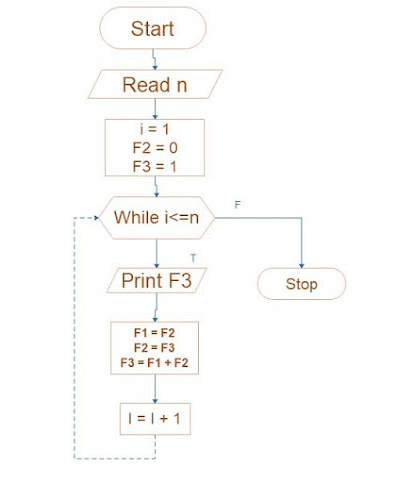# Algorithm Factorial and Fibonacci series - Ishwaranand

## Factorial

### Algorithm Factorial

• This is a flowchart to calculate the factorial of the given number.
• n-Accepted number
• fact-Factorial of number
• i - loop variable,Image Source ~ Crafted With ©Ishwaranand - 2020 ~ Image by ©Ishwaranand
step 1: Start
step 2: [Accepte a number] read n
step 3: set i=1, fact-1
step 4: Repeat steps 5 and 6 until i <= n
step 5: [calcute factorial] set fact=fact*i
step 6: set i=i+1
step 7: [ display factorial ] print fact
step 8: Stop

## Fibonacci series

### Algorithm Fibonacci

• This is the flowchart to display Fibonacci series for n terms
• n-Accepted number
• i-loop variable
• f1,f2,f3,- variable to manage Fibonacci seriesImage Source ~ Crafted With ©Ishwaranand - 2020 ~ Image by ©Ishwaranand
step 1: Start
step 2: [Accept a number of terms] read n
step 3: set i=1, f2=0,f3=1,
step 4: Repeat steps 5,6 and7until i<=n
step 5: [Display terms in series] print f3
step 6: set f1=f2, f2=f3, f3=f1+f3
step 7: seti=i+1
step 8: Stop